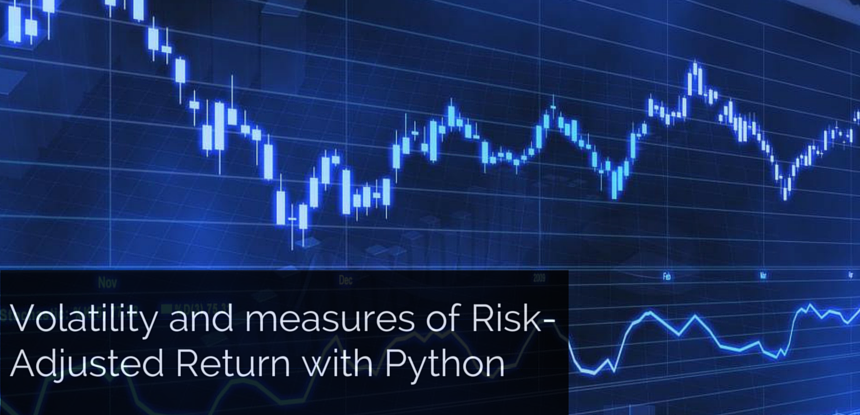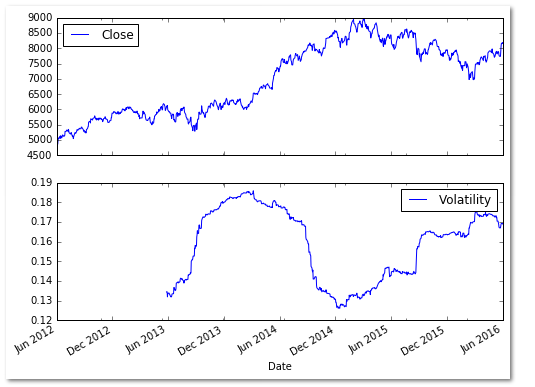# Volatility And Measures Of Risk-Adjusted Return With PythonIn this post, we see how to compute historical volatility in python, and the different measures of risk-adjusted return based on it. We have also provided the python codes for these measures which might be of help to the readers.

### Introduction

Volatility measures the dispersion of returns for a given security. It plays a key role in options trading. Volatility can be measured by the standard deviation of returns for a security over a chosen period of time. Historic volatility is derived from time series of past price data, whereas, an implied volatility is derived using the market price of a traded derivative instrument like an options contract.

#### Example: Computing historic volatility of Risk Adjusted Return for NIFTY

First, we use the log function from numpy to compute the logarithmic returns using NIFTY closing price and then use the rolling_std function from pandas plus the numpy square root function to compute the annualized volatility. The rolling function uses a window of 252 trading days. Each of the days in the selected lookback period is assigned an equal weight. The user can choose a longer or a shorter period as per his need.
```## Computing Volatility# Load the required modules and packages
import numpy as np
import pandas as pd
import pandas.io.data as web# Pull NIFTY data from Yahoo finance
NIFTY = web.DataReader('^NSEI',data_source='yahoo',start='6/1/2012', end='6/1/2016')# Compute the logarithmic returns using the Closing price
NIFTY['Log_Ret'] = np.log(NIFTY['Close'] / NIFTY['Close'].shift(1))# Compute Volatility using the pandas rolling standard deviation function
NIFTY['Volatility'] = pd.rolling_std(NIFTY['Log_Ret'], window=252) * np.sqrt(252)
print(NIFTY.tail(15))# Plot the NIFTY Price series and the Volatility
NIFTY[['Close', 'Volatility']].plot(subplots=True, color='blue',figsize=(8, 6))```### Measures of risk adjusted return based on volatility

#### Sharpe ratio

The Sharpe ratio which was introduced in 1966 by Nobel laureate William F. Sharpe is a measure for calculating risk adjusted return. The Sharpe ratio is the average return earned in excess of the risk-free rate per unit of volatility.
`Sharpe ratio = (Mean return − Risk-free rate) / Standard deviation of return`
Following is the code to compute the Sharpe ratio in python. The inputs required are the returns from the investment, and the risk-free rate (rf).
```# Sharpe Ratio
import numpy as np
def sharpe(returns, rf, days=252):
volatility = returns.std() * np.sqrt(days)
sharpe_ratio = (returns.mean() - rf) / volatility
return sharpe_ratio```

#### Information ratio (IR)

The information ratio is an extension of the Sharpe ratio which replaces the risk-free rate of return with the returns of a benchmark portfolio. It measures a trader’s ability to generate excess returns relative to a benchmark.

Following is the code to compute the Information ratio in python. The inputs required are the returns from the investment, and the benchmark returns.

```import numpy as np
def information_ratio(returns, benchmark_returns, days=252):
return_difference = returns - benchmark_returns
volatility = return_difference.std() * np.sqrt(days)
information_ratio = return_difference.mean() / volatility
return information_ratio```

#### Modigliani ratio (M2 ratio)

The Modigliani ratio measures the returns of the portfolio, adjusted for the risk of the portfolio relative to that of some benchmark. To calculate the M2 ratio, we first calculate the Sharpe ratio and then multiply it by the annualized standard deviation of a chosen benchmark. We then add the risk-free rate to the derived value to give M2 ratio.

Following is the code to compute the Modigliani ratio in python. The inputs required are the returns from the investment, benchmark returns, and the risk-free rate.
```# Modigliani Ratio
import numpy as np
def modigliani_ratio(returns, benchmark_returns, rf, days=252):
volatility = returns.std() * np.sqrt(days)
sharpe_ratio = (returns.mean() - rf) / volatility
benchmark_volatility = benchmark_returns.std() * np.sqrt(days)
m2_ratio = (sharpe_ratio * benchmark_volatility) + rf
return m2_ratio```

### Next Steps

Learn to optimize your portfolio in Python using Monte Carlo Simulation. This article explains how to assign random weights to your stocks and calculate annual returns along with standard deviation of your portfolio that will allow you to select a portfolio with maximum Sharpe ratio.

Update

We have noticed that some users are facing challenges while downloading the market data from Yahoo and Google Finance platforms. In case you are looking for an alternative source for market data, you can use Quandl for the same.

Disclaimer: The information in this project is true and complete to the best of our Student’s knowledge. All recommendations are made without guarantee on the part of the student or QuantInsti®. The student and QuantInsti® disclaim any liability in connection with the use of this information. All content provided in this project is for informational purposes only and we do not guarantee that by using the guidance you will derive certain profit.